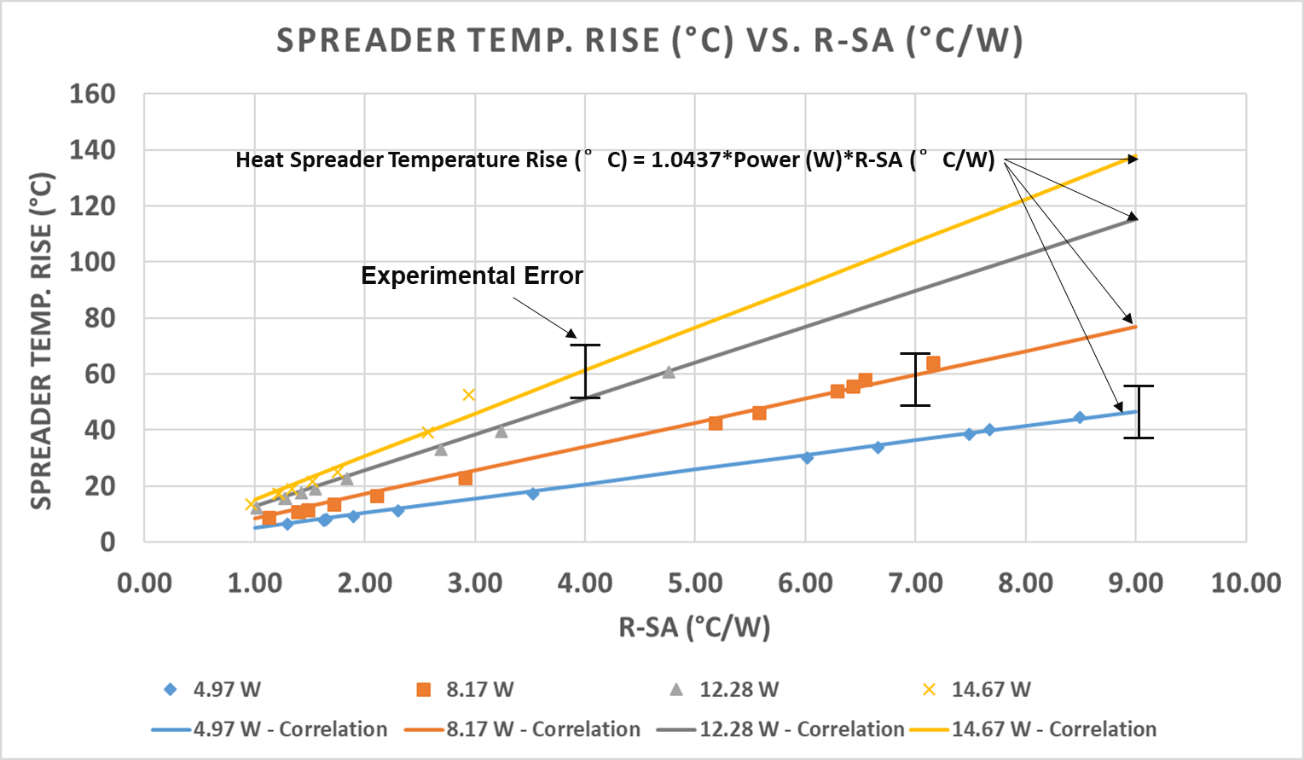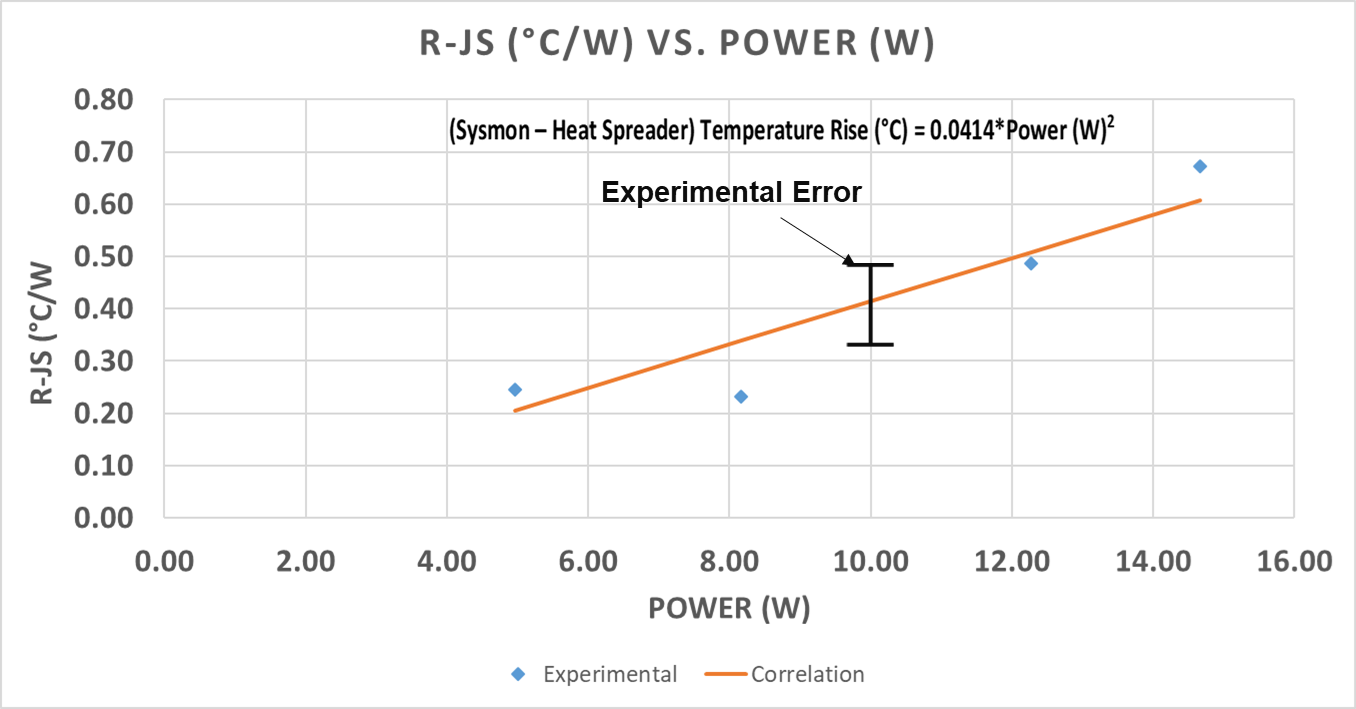# Empirical Correlations to Determine the Thermal Solution Performance

Document ID
UG1090
Release Date
2021-11-19
Revision
1.0 English

## Empirical Formula for the Heat Spreader Temperature Rise

The following empirical formula is used to calculate the heat spreader temperature rise:

Heat Spreader Temperature Rise (°C) = 1.0437 * Power (W) * R- SA (°C/W)
• Where,
• Heat Spreader Temperature = Ambient Temperature + Heat Spreader Temperature Rise
• R-SA is the thermal resistance of the cooling solution designed for your system, with TIM to ambient in °C/W.
• Power is the total SOM input power (W).

The following plot shows the deviation of the heat spreader temperature rise obtained from the above correlation for different input system powers associated to the experimental values. The points represent experimental data while the solid lines show the correlation trend which aligns very well with the test points.

Figure 1. Heat Spreader Temperature Rise vs. Thermal Resistance R-SAFrom this image you can use the correlation obtained from the estimated system power of your application and the target system thermal resistance of the solution installed on the K26 SOM heat spreader to find the heat spreader temperature rise.

## Empirical Formula to Find the Temperature Rise between the SYSMON and Heat Spreader

The following empirical formula is used to calculate the temperature rise between the K26 SYSMON and the heat spreader.

(SYSMON – Heat Spreader) Temperature Rise (°C) = 0.0414 * Power (W)2
Figure 2. Junction to Heat Spreader Temperature Rise vs. Total PowerUsing the correlation with the target system power, the expected temperature rise between the K26 SOM SYSMON and heat spreader can be calculated.

Note: The SYSMON temperature should always be less than the temperature specification given in Table 1.

## Illustrations of Applying the Empirical Correlations

In this first example, the system is operated at 35°C ambient, with a total estimated power of 15W and the thermal resistance of a cooling solution is 3°C/W. The SYSMON temperature is obtained by using the correlations outlined earlier in this section. Using the Empirical Formula for the Heat Spreader Temperature Rise:

Heat Spreader Temperature Rise (°C) = 1.0437 * Power (W) * R-SA (°C/W) = 1.0437 * 15 * 3 = 49.97°C
Heat Spreader Temperature (°C) = 49.97°C + Ta
Heat Spreader Temperature (°C) = 49.97°C + 35.00°C = 84.97°C
SYSMON Temperature (°C) = 0.0414 * Power (W)2+ Heat Spreader Temperature (°C) = 0.0414 * 152+84.97°C = 94.29°C

The result of this example is that with the estimated SYSMON temperature obtained from the empirical relations, you can verify whether your selected thermal solution meets the K26 SOM thermal specifications list in Table 1.

The second example has an estimated system power of 15W when operating in 25°C ambient temperature, the maximum SYSMON temperature is specified as 85°C. Using the correlations outlined earlier in this section, an estimated thermal resistance from heat spreader to ambient (R-SA) is calculated, defining an appropriate thermal solution as:

Heat Spreader Temperature (°C) = SYSMON Temperature (°C) – (0.0414 * Power (W)2) = 85 – (0.0414*152) = 75.69 °C
Heat Spreader Temperature Rise (°C) = 75.69°C – 25°C = 50.69°C
R-SA (°C/W) = Heat Spreader Temperature Rise (°C)/(1.0437 * Power (W)) = 50.69/(1.0437 * 15) = 3.2°C/W

## K26 SOM Standalone and Exposed to Air

In a K26 SOM system where the heat spreader is exposed to air, the recommended clearance above the heat spreader is approximately 30 mm. In this case, it is anticipated that the thermal resistance from the heat spreader to the ambient would be in the range of ~9 to 10°C/W. This arrangement can produce limited performance where the dissipated power from K26 SOM is smaller. It is crucial that K26 SOM is operating in a system arrangement that keeps the SYSMON temperature below the specification outlined in Table 1.

For example, a K26 SOM with a heat spreader that has a thermal resistance from the spreader to an ambient temperature of 9°C/W. The area surrounding the K26 SOM in 30 mm of free area is 35°C, and the maximum SYSMON temperature specified from the specification is 100°C. Under this condition, the maximum dissipated power from the K26 SOM is described in the following formulas.

Heat Spreader Temperature – Ambient temperature (°C) = 1.0437 * Power (W) * R-SA (°C/W) = 1.0437 * Power * 9
Heat Spreader Temperature – 35 (°C) = 9.39 * Power
SYSMON Temperature (°C) – Heat Spreader Temperature (°C) = 0.0414 * Power (W)2
100 (°C) – Heat Spreader Temperature (°C) = 0.0414 * Power (W)2

Calculating the sum of the two equations:

65 (°C) = 9.39 * Power +0.0414 * Power (W)2

Solving this equation gives Power = 6.8W.

Thermal Tip: Following best engineering practices includes numerically modeling the parameters using the thermal models provided by Xilinx. The numerical model must be validated with experimental data to ensure that the K26 SOM system is operated under the rated specifications as per these guidelines and the data sheet. The empirical correlations provided in this document are only for an initial thermal assessment and cannot be used for production thermal design.